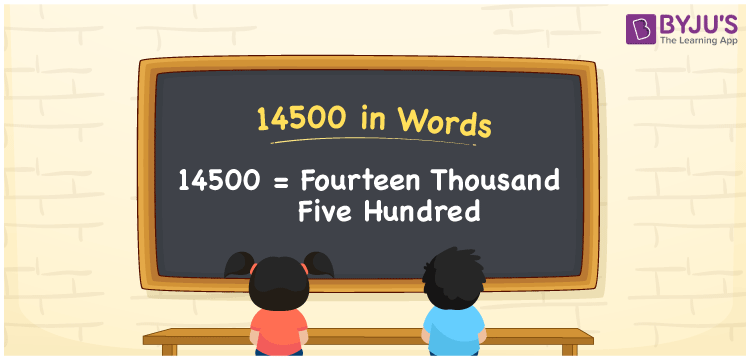# 14500 in Words

14500 in words can be written as Fourteen thousand five hundred. In the article 14500 in words, students will get a clear cut idea of counting numbers. For example, if you purchase a laptop for Rs. 14500, then you can say that “I purchased a laptop for Fourteen thousand five hundred rupees”. You will understand how to convert the number 14500 into words in this article. English alphabets are made use of while writing the numbers in words. So, 14500 can be read as “Fourteen Thousand Five Hundred” in English.

 14500 in words Fourteen Thousand Five Hundred Fourteen Thousand Five Hundred in Numbers 14500

## 14500 in English Words## How to Write 14500 in Words?

Here you will learn how 14500 can be written in words. Five digits are present in the number 14500. You can learn the place value chart for all the five digits of the number 14500 from the table provided below.

 Ten thousands Thousands Hundreds Tens Ones 1 4 5 0 0

The number 14500 can be expanded in the form:

1 x Ten thousand + 4 × Thousand + 5 × Hundred + 0 × Ten + 0 × One

= 1 × 10000 + 4 × 1000  + 5 × 100 + 0 × 10 + 0 × 1

= 10000 + 4000 + 500

= 14500

= Fourteen Thousand Five Hundred

Hence, 14500 in words is written as Fourteen Thousand Five Hundred.

14500 is a natural number that precedes 14501 and succeeds 14499.

14500 in words – Fourteen Thousand Five Hundred

Is 14500 an odd number? – No

Is 14500 an even number? – Yes

Is 14500 a perfect square number? – No

Is 14500 a perfect cube number? – No

Is 14500 a prime number? – No

Is 14500 a composite number? – Yes

## Frequently Asked Questions on 14500 in Words

Q1

### How can 14500 be written in words?

14500 can be written in words as “Fourteen thousand five hundred”.
Q2

### Is 14500 an odd number?

No, 14500 is not an odd number as it is divisible by 2.
Q3

### How Fourteen thousand five hundred can be written in numbers?

Fourteen thousand five hundred can be written in numbers as 14500.